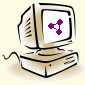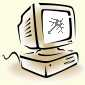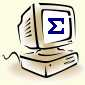## Programs

These programs (Windows applications) are included on a CD-ROM with the book. You may also download them here.Axelrod Axelrod implements a genetic algorithm to find optimal strategies for playing iterated prisoner’s dilemma.Benford Benford calculates significant-digit occurrences in a set of data and compares their distribution with Benford’s law.Bernoulli Bernoulli calculates the occurrences of continuous blocks of 1s of different length, within a sequence of 0s and 1s.Bertrand Bertrand illustrates the famous Bertrand paradox.Buffon Buffon uses a pseudo-random number generator to simulate the successive tossing of a coin and calculates statistics.Cantor Cantor allows designing and rendering custom two-dimensional self-similar fractal objects.Conway Conway implements the evolution of user-defined two-state two-dimensional cellular automata on a square, triangular, or hexagonal lattice.Ehrenfest Ehrenfest simulates the dogs-and-fleas process described by Paul Ehrenfest.Euler Euler finds the shortest Hamilton cycle within a given graph.Feigenbaum Feigenbaum plots a map of the chaotic behavior of the logistic (or user-defined) function.Galton Galton simulates the behavior of a Galton board to produce a Gaussian distribution.Holland Holland attempts to find the maximum of a given function using a genetic algorithm.Hopfield Hopfield uses a neural network to learn the user’s handwriting and to then recognize characters written in the user’s hand.Huffman Huffman constructs an optimal questioning strategy tree given a distribution of probabilities and uses this algorithm to compress and decompress files.Hyman Hyman allows conducting experiments which show that human response times to varied signals are proportional to the information content of these signals.Lorenz Lorenz illustrates the butterfly effect by modeling a meteorological phenomenon susceptible to chaotic behavior.Mandelbrot Mandelbrot allows examining the Mandelbrot set, Julia sets, and Newton’s fractal.Pasteur Pasteur implements a cellular automaton for modeling the spread of a disease within a population of organisms.Poe Poe makes a statistical analysis of a given text and uses it to determine the language the text is written in.Schelling Schelling uses a simple cellular automaton to simulate racial segregation within a society.Shannon Shannon calculates the information entropy, given a distribution of probabilities.Sierpinski Sierpinski draws self-similar fractal images by means of a two-dimensional random walk.Smoluchowski Smoluchowski simulates the Brownian motion of a group of particles in one, two, or three dimensions.Turing Turing emulates the behavior of a user-defined Turing machine.Ulam Ulam uses the Monte Carlo method to estimate the integral of a given function in a given range.

Polska wersja | Home | Overview | Contents | Programs | Errata | Buy online | Numeric files | Links | Cover | Contact